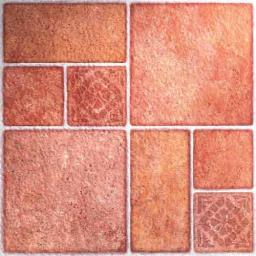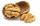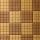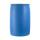# Tiles

From how many tiles 20 cm by 30 cm, we can build a square of maximum dimensions if we have maximum 881 tiles.

n =  864

### Step-by-step explanation:Did you find an error or inaccuracy? Feel free to write us. Thank you!Tips to related online calculators
Do you want to calculate least common multiple two or more numbers?
Looking for help with calculating roots of a quadratic equation?

#### You need to know the following knowledge to solve this word math problem:

We encourage you to watch this tutorial video on this math problem:

## Related math problems and questions:

• TilesHow many tiles of 20 cm and 30 cm can build a square if we have a maximum of 100 tiles?
• PlumberThe plumber had to cut the metal strip with dimensions 380 cm and 60 cm to the largest squares to no waste. Calculate the length of the sides of a square. How many squares cut it?
• NutsHow many we must have at least nuts if we can equally divide it to 10 children, 12 children or 15 children and any nut left?
• Cuboid wallsCalculate the cuboid volume if its different walls have an area of 195cm², 135cm², and 117cm².
• Square roomWhat is the size of the smallest square room, which can pave with tiles with dimensions 55 cm and 45 cm? How many such tiles is needed?
• Uboid volumeCalculate the cuboid volume if the walls are 30cm², 35cm², 42cm²
• Bricks pyramidHow many 50cm x 32cm x 30cm brick needed to built a 272m x 272m x 278m pyramid?
• RectangleThe perimeter of the rectangle is 22 cm and content area 30 cm2. Determine its dimensions, if the length of the sides of the rectangle in centimeters is expressed by integers.
• TilesHow many square tiles with an area of 121 cm2 have to be ordered for the square room's paving with a side length of 2.75 meters?
• TilesHall has dimensions 325 &time; 170 dm. What is the largest size of square tiles that can be entire hall tiled, and how many we need them?
• A number 5A number is divisible by 24, 25, and 120 if it is increased by 20. Find the number.
• SquareSuppose the square's sides' length decreases by a 25% decrease in the content area of 28 cm2. Determine the side length of the original square.
• Wooden bowls20 wooden bowls in the shape of a truncated cone should be painted on the outside and inside with wood varnish. We need 0.1 l of paint to paint 200 cm2. How many liters of paint do we have to buy if the bowls are 25 cm high, the bottom of the bowl has a d
• Bricks wallThere are 5000 bricks. How high wall thickness of 20 cm around the area which has dimensions 20 m and 15 m can use these bricks to build? Brick dimensions are 30 cm, 20 cm and 10 cm.
• Triangle SASCalculate the triangle area and perimeter, if the two sides are 51 cm and 110 cm long and angle them clamped is 130 °.
• TrapezoidArea of trapezoid is 135 cm2. Sides a, c and height h are in a ratio 6:4:3. How long are a,c and h? Make calculation...
• TankIn the middle of a cylindrical tank with a bottom diameter 251 cm is standing rod which is 13 cm above the water surface. If we bank rod its end reach surface of the water just by the tank wall. How deep is the tank?